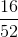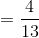# High School Math : Understanding Number Sets

## Example Questions

### Example Question #6 : Number Theory

If a card is drawn randomly from a regular shuffled 52 card deck, what is the probability that the card is either a spade or a 3?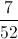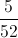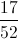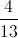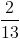Explanation:

How many cards in the deck are either a spade or a 3?

There are four 3's, including a 3 of spades.

Since we are counting the same card (3 of spades) twice, there are actually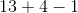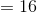distinct cards that fit the criteria of being either a spade or a 3.

Since any of the 52 cards is equally likely to be drawn, the probability that it is a spade or a 3, is